# Updates on "Coloring graphs using topology" and "Graphs with Eulerian Unit spheres"

This is a mini blog, commenting on some updates of the preprint.
1.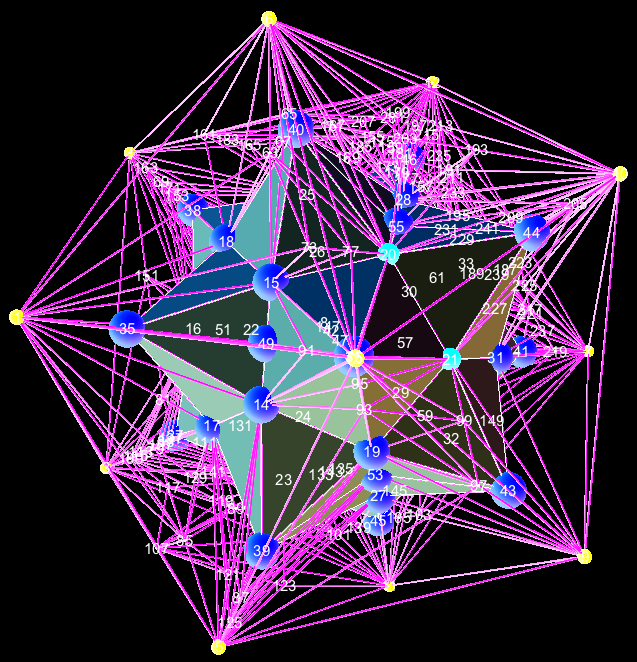March, 2015 (Spring break) Still programming on automatic cutting.
2.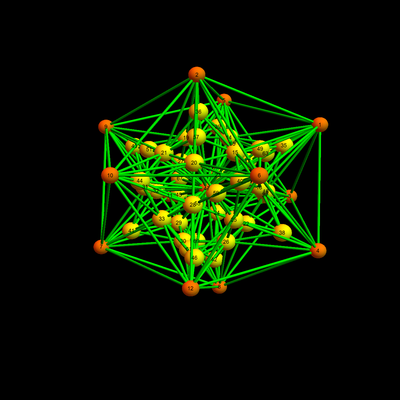February 1, 2015: A lot of heavy programming still needs to be done. Obstacles are expected to remain. Here are some pages of a research diary:  (sketch of main idea), , , , , , , , , , , , , , , , (last entry March 27, 2015). We will use that it is possible to make Eulerian any two sphere, on which a open disc and additionally a subset of a triangle is not to be touched for cutting. Given a 2-sphere graph G, we want to build an Eulerian 3-ball to which G is a boundary. We first pad G with a central sphere G' and an additional central point to have a 3-ball of radius 2 in which an Eulerian cave. Here is a picture, where an icosahedron has been padded first by an inner completed dual sphere, then stellated with an inner vertex so that the icoshedron is now the boundary of a ball of radius 2. The goal will now be to increase the Eulerian inner part until everything is Eulerian. The key is not to cut in parts which are already cleaned out. The programming part which remains looks heavy and there could also be fundamental obstacles. Two important steps need still to be realized in the computer: the procedure which cleans out a 2-sphere with obstructions and then to apply this "knife" which makes the surgery at the inner spheres in an automatic way. This needs to be done efficiently, as otherwise, we end up already for a small graph with a 3-ball having thousands of tetrahedra. (We expect for a graph G of order n to have a in n polynomial number of tetrahedra in the Eulerian final ball B which has G as the boundary). Unlike in previous work, the cleaning of the two sphere was now already done automatically by the computer. We do no more have to tell which edges to cut. The computer did this already automatically and deterministically. In this picture, the central vertex has already been cleaned so that the most inner sphere is Eulerian and all the edges to the most inner node have now even degree. Now, we will have to start cleaning out the unit spheres of vertices located on the most inner sphere etc. This will increase the number of nodes but we do not touch edges of the inner sphere any more. In general, we do not touch the open part which has already been rendered Eulerian like that. The end game, where we have only very few edges left to clean, we rely on the fact that on a 2 sphere, the odd degree vertices can never be supported on a subset of a triangle only. As unit spheres of inner vertices will always intersect the boundary graph G maximally in a triangle, we insist also to be able to do the cutting under the handicap of not touching edges of a triangle. But the automatic cleaning algorithm does not care about such a triangle (the geodesic paths along which the cutting is done can jump over it. This is already implemented). One can be worried that the cutting will dig us in. As more we cut, as more vertices will be added, whose unit spheres will have to be cleaned: the cleaning could become a Sisyphus work. But the cutting procedures do not change the radius of the ball and we have a definite bound on the number of unit spheres which need to be cleaned: it is the number of inner nodes in this picture. If this is right, then the entire algorithm is expected to cost less than a constant times n times the maximal size of a unit sphere of a vertex on the central unit sphere. This looks quadratic in n and if one could bound the maximal size of unit spheres in B of vertices on the inner unit sphere, be even linear.
3.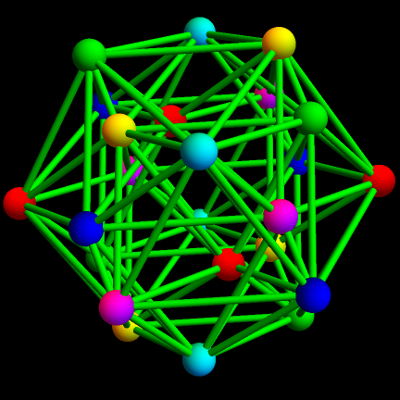January 12, 2015: More details on the geometry of graphs which can be colored minimally: "Graphs with Eulerian Unit spheres" and local copy. It was important to write and get this out quickly due to a backlog of other (mostly non-mathematical) things to do. This was written during the winter break and had therefore the deadline January 12. The paper gives a crystal clear definition of what a sphere is in graph theory as mentioned here already, essentially characterizes minimally colorable spheres and relates them with an important subclass of graphs on which we can run a unique geodesic flow. (which can be run on a computer for any network with the right properties). We define
 Platonic d-spheres G are d-spheres for which all unit spheres of G are isomorphic to a single Platonic (d-1)-sphere H.
and give a full classification using the Gauss-Bonnet-Chern result in graph theory only; using only the induction assumption that the empty graph is the (-1)-sphere. The missing polytopes are not spheres in our case as their unit spheres are not spheres. For the 24-cell for example, seen to the right, all unit spheres are cube graphs. And the cube graph itself not a sphere because removing a point does not make it collapsable. It's amazing that with such a simple inductive definition, starting with the assumption that the empty graph is a platonic (-1)-sphere, one can get to almost all higher dimensional regular polytopes.January 3 2015: The book "Geloeste und ungeloeste Mathematische Probleme aus alter und neuer Zeit" by Heinrich Tietze was known to me since high school. I liked these type of books very much. They were probably a reason, why I started to study mathematics. Tietze's work a 2 volume book written for a general audience, based on lectures given for a general audience. And as the book clearly indicates, "for a general audience" does not mean to trivialize or refrain from questions actually pursued by mathematicians. There are two chapters which are essentially dedicated to coloring problems. Tietze had worked himself on graph coloring and especially dealt with the problem to find the chromatic number of the Moebius strip (figure in the book). Tietze wrote in that book on page 66-67:
 "Die Mutmassungen der Mathematiker, ob sich einmal die "chromatsche Zahl" für Ebene und Kugelflache gleich 4 oder gleich 5 ergeben wird, sind geteilt und baben auch bei manchem Forscher wahrend der Beschäftigung mit dem Problem geschwankt. Ob Aussicht besteht, dass neue, die Lösung der Frage erzwingende Üerlegungen sich auch von allgemeinerer Bedeutung erweisen und nicht nur dem speziellen Kartenfarbe-Problem zugute kommen werden, mag dahingestellt bleiben. Im letzteren Fall würde unser Problem gleichwohl in der Geschichte der Mathematik einen Platz behalten als eine jener Fragen, an denen das Unwesentliche weitgehender Gestaltsänderungen, d.h. der topologische Charakter der Aufgabe, deutlich erkennbar ist, - somit als ein interessanter Vorläufer der Topologie. Im übrigen aber, wenn die Lösung einmal auf harmlose Weise gelingt, dann versinkt unser Problem vielleicht inmitten anderer aus vergangenen Zeiten aufgespeicherter spezieller Aufgaben. Vielleicht aber gehen von ihm noch neuartige Methoden aus, die ein schöpferisches Genie zu seiner Lösung ersann und durch die neuen Entwicklungen die Wege gebahnt werden. Wer kann das wissen?" "The opinions of mathematicians, whether the chromatic number for the plane or sphere is 4 or 5 are divided; many of the researchers working on the problem might have changed them over time. Whether new considerations will force the solution to be of general importance or only useful for in the particular case of the four color theorem is not clear. In the later case, the problem will still have a place in the history of mathematics as a problem for which the topological character of the problem is visible and remain an interesting precursor of topology. If the solution will be found using innocent methods, our problem might sink into the class of other special problems accumulated over time. Maybe however, other new methods will emerge from it, which a creative genius imagines in order to solve the problem and through which new developments will emerge. Who can know?"

Tietze was a gifted teacher. As the biography of Heinrich Heesch indicates, Tietze was among Perron, Caratheodory and Hartogs a teacher of Heesch. There is a citation of Aumann about Tietze:

 Tietzes Vorlesungen waren wegen ihrer Klarheit und Eindringlichkeit bei den Studenten hoch geschätzt. Seine Seminare waren als grosse Übungen in geistiger Disziplin bekannt." The lectures of Tietze were highly appreciated by students because of their clarity and intensity. His seminars were great exercises in mental discipline.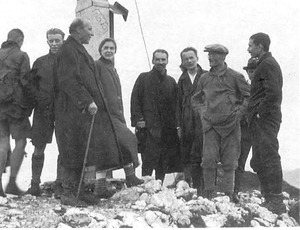Here is a photo (click for larger version) taken in the summer of 1927 from the book on Heesch showing Caratheodory (with stick), Elli Heesch (the sister of Heinrich), Heinrich Tietze, (looking a bit like Kevin Klein), Heinrich Heesch, Fritz Lettenmeyer (with hat, a student of Lindemann and assistant of Perron) as well as other students. (I believe the hidden person, second from the right looking down is Oscar Perron, who was almost a fanatic mountain climber). The mountain could be the Steckbrief but I could not match the shape of the "Gipfelkreuz" reliably with any of these. [Jan, 4 2015: after having seen this and this, the mountain could be the "Grosse Traithen", also since Sommerfelds lodge was nearby]. As Perron recalls, (ref: Heesch's biography), Tietze was the organizer of many mountain tours: instruct students even during seminars about good shoes and clothing and make test tours with an assistant before.Also a figure in Heesch's biography. It shows Karl Selmayer, the mechanics of Sommerfeld, Arnold Sommerfeld and Heesch in 1926. Funny how they were wearing ties even while skiing. Sommerfeld is my own academic grand4 father. By the way, I still used as a student the book of Sommerfeld in the 3rd semester mechanics course given by Juerg Froehlich. Froehlich's lectures were however closer to Arnold's book "Mathematical methods of classical mechanics". Heesch had been a student of Sommerfeld in his course "dynamical problems in atomic physics" and been asked by Sommerfeld to work out his notes. In total, Heesch worked out notes for 5 courses for Sommerfeld. The families of Sommerfeld and Heesch were close as they were playing music together. Sommerfeld had a little cabin in the Wendelstein mountains in the Bavarian alps, so that the best guess is that this picture of 1926 has been taken nearby. Again, click to see the larger scan.
4. January 3: a tiny adaptation of the inductive definition given in the slides [PDF]: it is better not to define the class B-1 and leave S-1=G-1 to be the class of graphs containing only the empty graph. There are various reasons for that: first of all, the class of spheres and balls are then always different. Naturally, the empty graph has Euler characteristic 0. With this assumption, it is always true that spheres have Euler characteristic 1+(-1)d and it remains especially true in dimension d=-1, where the empty graph is considered a sphere and the only graph of dimension -1. Also, what is now always true is that graphs in Bd have Euler characteristic 1, for all d ≥ 0. It is also true that one could define for d ≥ 1, the class Bd of d-dimensional balls without referring to spheres: define first B0 as the set of graphs containing the one point graph only, then define the class Bd as the class of contractible graphs of dimension d for which each punctured unit sphere is in Bd-1. This is nothing different, its just bypassing the name "sphere" and illustrates a bit better that the construction of an Euclidean notion in graph theory only needs homotopy and dimension, similarly as it does in the continuum as indicated here: lets look at the class Bd of metric spaces in an inductive way. For d=0, it is the metric space containing only one point. The class Bd is now defined for d ≥ 1 as the class of metric spaces such that every small enough punctured sphere Sr(x) (points in distance r from x) is in Bd-1. This forces B1 to be the class of open intervals and then B2 to be the class of two-dimensional topological manifolds homeomorphic to the open unit disc and Bd to be the class of three dimensional manifolds homeomorphic to the open unit ball in R3.
5. December 27, 2014: Some slides [Youtube]. [PDF]. Added January 3, 2015: The slide on the Kantons of Switzerland [PNG] was scanned by me from the original book of Tietze. Of course the topology has changed since Tietze wrote his book as the Kanton of Jura separated from Bern in 1979. Also, Tietze simplified the situation a bit by not separating Appenzell, Basel Nid-Obwalden into two cantons as officially done. And then there are enclaves like of Solothurn or Schaffhausen. Today there are 26 cantons. The simplified map of Tietze shows 22 cantons. Tietze's Switzerland graph has dimension 998/495=2.01414, which is 1 plus the average of the dimensions of unit spheres: (13/9, 2, 19/15, 2, 1, 1, 1, 0, 4/5, 1, 1, 1, 1, 1, 4/5, 1, 1, 1, 0, 1, 1, 1). The Kantons of Geneva and Appenzell have dimension 1 as their unit spheres consist of one point only. The Switzerland graph of Tietze is still contractible and has Euler characteristic 1 and Betti vector (1,0,0,0) and Poincaré polynomial p(x)=1. The simplex data are v=(22,45, 25, 1) so that the Euler polynomial is e(x) = 22+45x+25x2+x3. The identity e(-1)=p(-1) is the Euler-Poincarée identity which holds in full generality for all finite simple graphs. The graph contains one tetrahedron (1,3,4,14) only, which is formed by the cantons of Basel,Bern,Aarau. and Solothurn. This tetrahedron is the reason why the actual dimension is slightly larger than 2 despite having some points of dimension lower than 2. The chromatic polynomial of the Switzerland graph of Tietze is c(x) = (x-3)3(x-2)7(x-1)3x(8-5x+x2) (281-583x+538x2-278x3+84x4-14x5+x6). There are c(4) = 1603584 possible colorings with 4 colors which means that for every 5 people in Switzerland, there is a coloring. Already the presence of a tetrahedron prevents a 3 coloring but there are also cantons with an odd cyclic unit sphere like Schwyz (with a cyclic graph C7 as a unit sphere needing 3 colors already) so that the unit ball centered at Schwyz already needs 4 colors.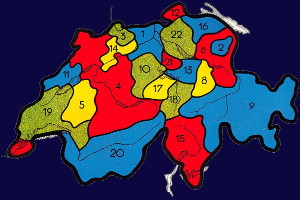Picture from Tietze's book. Click for a larger picture. Tietzes book was printed with 3 colors so that he had to dither the green color.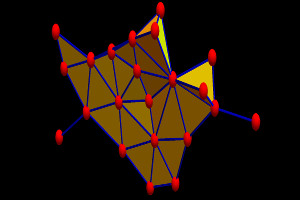Drawn with Mathematica with triangles filled. The shape of Switzerland is usually compared with a pig. This graph looks more like a humming bird. Click for a larger picture.Refining a triangulated three dimensional sphere (the tesseract).
6. Some illustrations with explanations [HTML] containing some 1200x1200 pixel figures.
7. A Youtube movie clip explaining the cutting game. If one could show that every three dimensional, simply connected graph can be subdivided so that all interior edge degrees become even, then one would prove the 4 color theorem. The game is a bit like the well known Boolean lights out game but it is more difficult as every switching step makes the game larger. Playing poorly at the beginning produces a larger and larger mess which is harder to solve. I have implemented the game on the computer but Mathematica takes more than a minute to compute the new situation (as I use general packaged procedures, computing for example every time from new all the tetrahedra in the graph etc). January 3, 2015: Having spent most of my time programming that stuff since the summer, I'm reluctant currently to reprogram that from scratch especially since the math is also interesting. The best would be to start from scratch, programming the game in C. A mathematical approach would completely disregard the geometry and implement things as a Gaussian elimination game where every elimination step adds more rows and columns. Still, the reason why the game can be won is topological: take a closed path and remove it by finding a surface which has the path (as well as part of the boundary of the entire graph) as boundary. Slicing along this surface then removes that closed path from the list of edges with odd degree. Then move to the next closed path.
8. The main coloring idea (can also be seen as a game). Subdivide the interior until all edge degrees are even. Then we have colored the outside. Here is the 4 color game: its quite challenging, even for a computer. But it does the job and colors the planar graph with 4 colors in the end (if one can always win this game, then this is a proof of the four color theorem):9. December 24: Ivan Izmestiev from the Arbeitsgruppe Diskrete Geometrie was kind to send me some articles relevant to the topic. It will be incorporated into the preprint (the local version is kept up to date).
• The result that a triangulation of the sphere is 4 colorable if and only if can be extended to an even triangulation of the ball B3 has been discovered independently by several mathematicians in the late 70'ies: it is the following theorem (this is mentioned and generalized to higher dimensions in Izmestiev's paper of 2005 in the European Journal of Combinatorics). Given a sphere triangularization. It is 4 colorable if and only if it can be extended to a 4 color triangulation of the ball B3, where every inner edge is surrounded by an even number of tetrahedra. Like Josmig and Izmestiev, who rediscovered this in 2003, Jenny and I rediscovered it in our summer project 2014 too. Fisk showed that for any G which is d+1 colorable and which is the boundary of a d+1 dimensional graph H, the coloring can be extended to H. What appears still to be new is that we take the cobordism idea extremely seriously as a computation tool for coloring and possibly (in the future) for a proof of the four color theorem. As usual, the implementation of the structures on the computer took the bulk time of this research, where it helped that I have written a graph theory library in Mathematica which is now nearly 10'000 lines of tightly programmed code (of course the bulk is taken by examples and various illustration code). The actual computations usually only take a few lines which makes it very inefficient (but easy to program as we deal with well separated computation units which can be tested independently). The above animation takes about an hour to render since I do not yet compute things in an optimized way (and in every step for example compute newly a list of all the tetrahedra in the graph). Written from scratch in C, this would take a fraction of a second or could be rendered in real time. It actually produces nice puzzles: take a graph which triangulates the sphere. The player has to subdivide the interior of the ball to render each edge even. Once done the graph is 4 colored. We have played this game for a while now: one has to start clearing out the inside of course as otherwise, the subdivisions outside make it difficult to see whats going on in the interior.
• Related to the Fisk torus example is an article of 2013, where Izmestiev, Kusner, Rote, Springborn and Sullivan show for example that a 1/6,-1/6 curvature pair can not be realized alone on a torus. Here is a figure from their article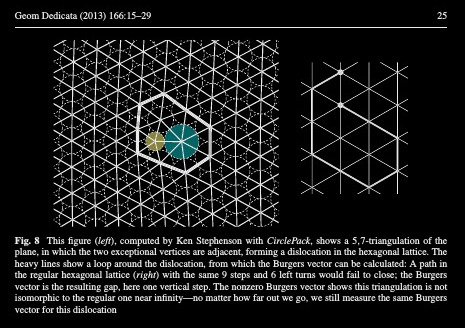Its like the curvature pair produces a "ripple" in space which is still visible in form of a holonomy at infinity. It can not be slapped onto a torus. Very much like in solid state physics or quantum mechanics (Ahoronov-Bohm).
10. December 23: Found work of Bojan Mohar on the projective plane and Klein bottle supporting the claim. The local copy of the paper has an update Also corrected some misprints and a statement in figure 28 which still was there before learning about the Fisk construction.